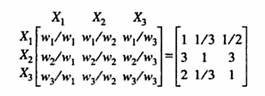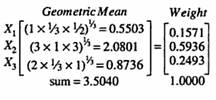# Converting ratings into importance weights

January 18, 2011
Respondents were asked to rate the various factors on a scale. These ratings were converted into importance weights (percentage weights summing up to 100 per cent).

A respondent assesses n(n-1)/ 2 importance ratios between attributes. This information is stored in the upper (or lower) triangle of a (n x n) matrix, whose typical element ajk represents the weight ratio of wi/wk. The remaining elements of the matrix are filled by employing the reciprocal of the matrix: ajk = 1/akj and ajj = 1, for all j and k.

Example: The following pair wise judgments were madeWe compute the geometric mean of each row of the matrix and then normalise the resulting numbers:The ratings of each respondent are converted into weights. For a given segment of the sample of respondents, the mean weights for every factor are then computed.

 URL for this article : https://www.businesstoday.in/magazine/best-companies-to-work-for/converting-ratings-into-importance-weights/story/12519.html @ Copyright 2019 India Today Group.